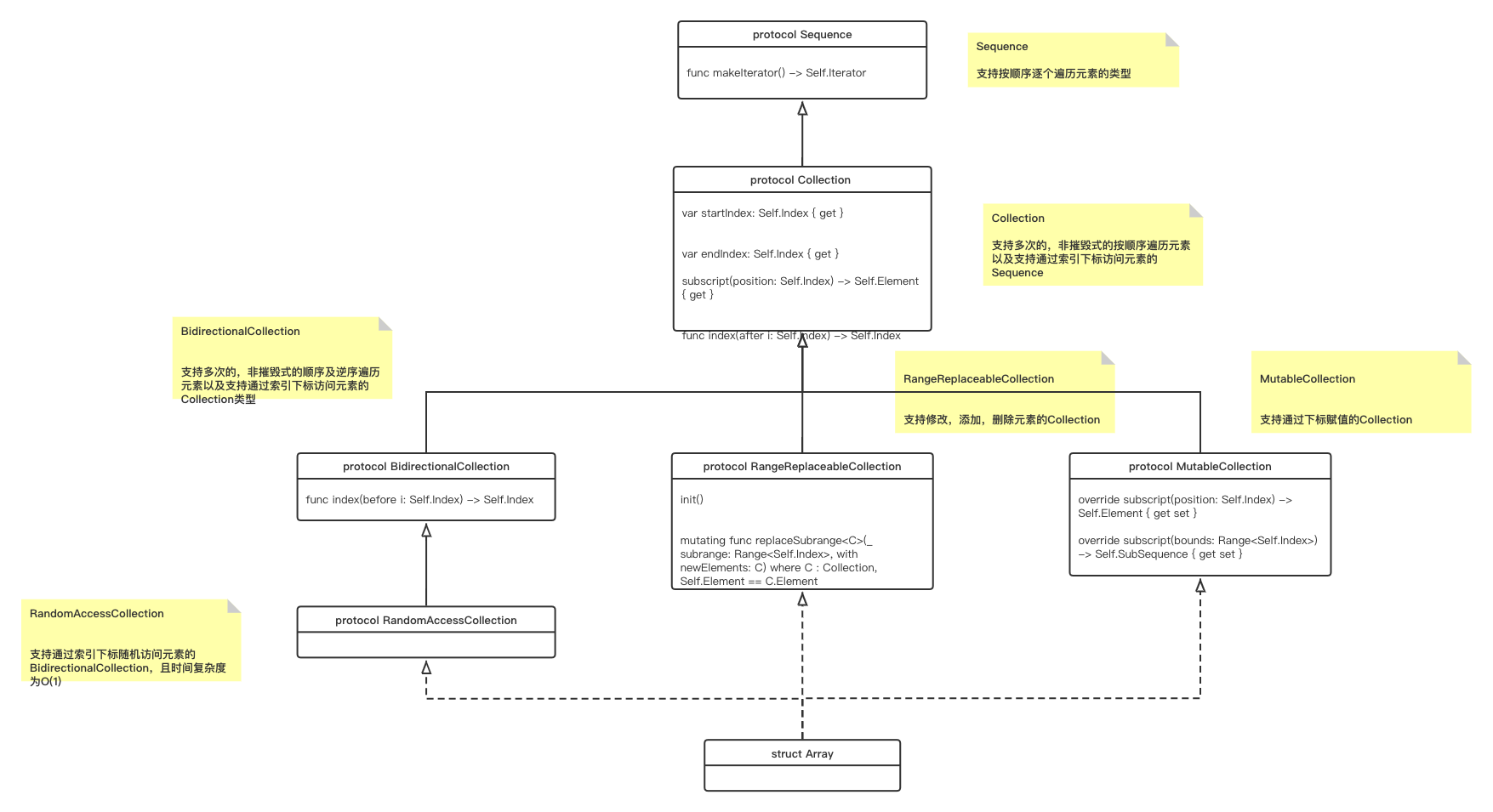# Array

`Array` 是有序的，支持随机读写的集合类型
@frozen struct Array<Element>## 1. 基本操作

### 1.1 初始化，构造

// 创建空的Array, 类型推断
let array1 = [Int]()
var array2 = Array<Int>()
// 创建空的Array, 指定类型
var array3 : [Int] = []
// 通过列表初始化
var array4 = [1,2,3,4,5]
// 调用init方法初始化
var array5 = Array(repeating:0, count:3)

### 1.2 增加元素

`+``append(_:)` 可以在数组尾部添加元素
`insert(_:,at:)` 在索引指定的位置插入元素
array1.append(11)
array1 += [13,12]
array1.insert(13,at:0)

### 1.3 删除元素

`remove(at:)` 删除索引指定位置的元素
`removeLast()` 删除数组中的最后一项
//
array1.remove(at:5)
// Can't remove last element from an empty collection:
array1.removeLast()

### 1.4 访问数组

array1
array1 = 12
// 下标法可以使用区间运算符
array1[1...3] = [2,3,4]

### 1.5 遍历数组

for value in array1 {
print("\(value)")
}

for (index, value) in shoppingList.enumerated() {
print("Item \(String(index + 1)): \(value)")
}

## 3. 修改Array 的拷贝

Array 是struct类型，也就是值类型。Array对象的每一个拷贝之间都是独立的，修改一个拷贝并不会影响其他拷贝。

var numbers = [1, 2, 3, 4, 5]
var numbersCopy = numbers
numbers = 100
print(numbers)
// Prints "[100, 2, 3, 4, 5]"
print(numbersCopy)
// Prints "[1, 2, 3, 4, 5]"

// An integer type with reference semantics
class IntegerReference {
var value = 10
}
var firstIntegers = [IntegerReference(), IntegerReference()]
var secondIntegers = firstIntegers
// Modifications to an instance are visible from either array
firstIntegers.value = 100
print(secondIntegers.value)
// Prints "100"
// Replacements, additions, and removals are still visible
// only in the modified array
firstIntegers = IntegerReference()
print(firstIntegers.value)
// Prints "10"
print(secondIntegers.value)
// Prints "100"

var numbers = [1, 2, 3, 4, 5]
var firstCopy = numbers
var secondCopy = numbers
// The storage for 'numbers' is copied here
numbers = 100
numbers = 200
numbers = 300
// 'numbers' is [100, 200, 300, 4, 5]
// 'firstCopy' and 'secondCopy' are [1, 2, 3, 4, 5]

## 4. Array 和 NSArray 之间桥接

`colors``Array<String>`的数组，元素类型为 `String`, `String` 是一个Foundation类型，可以桥接；
`moreColors``Array<String?>`, 元素类型为 `String?`, `String?` 本质上是枚举类型，不是`class`或者`@objc protocol`或者`Foundation类型`中任意一种，因此不可以桥接。
let colors = ["periwinkle", "rose", "moss"]
let moreColors: [String?] = ["ochre", "pine"]
let url = URL(fileURLWithPath: "names.plist")
(colors as NSArray).write(to: url, atomically: true)
// true
(moreColors as NSArray).write(to: url, atomically: true)
// error: cannot convert value of type '[String?]' to type 'NSArray'